# Assignments

The integrals below can be calculated by using a special method: integration by parts. For some assignments different techniques have to be applied. Always verify the result.

1. Calculate: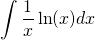Solution

2. Calculate: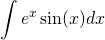Solution

3. Calculate: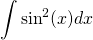Solution

4. Calculate: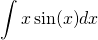Solution

5. Calculate: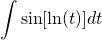Solution

6. Calculate:Solution

7. Calculate: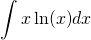Solution

8. Write the following integral in a recursive form: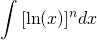Solution

9. Show the following formula (we have already applied this a number of times):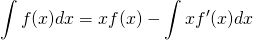Solution

10. In assignment 3 it was asked to calculate the following integral and we applied integration by parts. Is there another way and if so, which?Solution

0